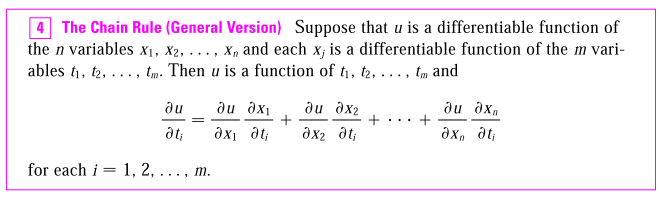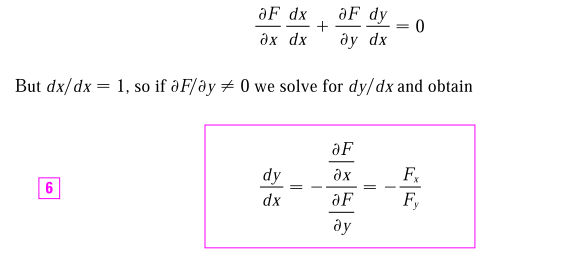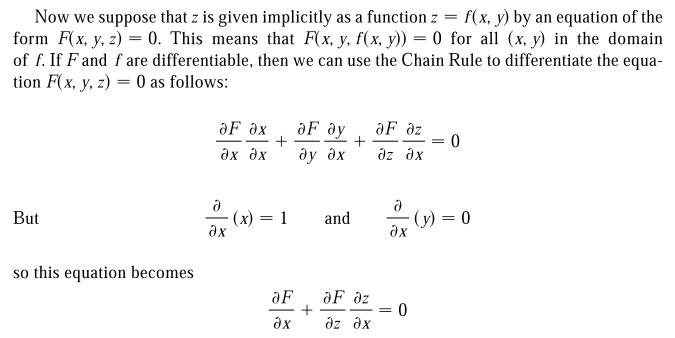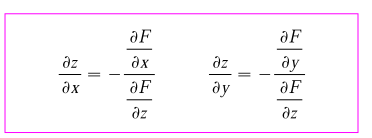## The Multivariable Chain RuleAn application of chain rule:Note the positions of $x$ and $y$ changed on the right hand side. You can also see it this way:

\begin{align*} \frac{dy}{dx} &= -\frac{\partial y / \partial F}{\partial x / \partial F} \\ \end{align*}

More generally:You can also see it from a differential form's point of view.

\begin{align*} g(x, y, z) &= c \\ g_xdx + g_ydy + g_zdz &= 0 \\ \end{align*}

Remember the definition of $\partial z / \partial x$ is the rate of change of z with respect to x when y is constant, i.e. $dy = 0$, the rest follows easily.